Anzeige

# MATH 6 PPT Q3 – Translation Of Real-Life Verbal Expressions And Equations Into Letters Or Symbols.pptx

28. Mar 2023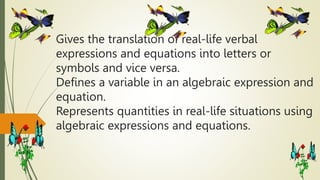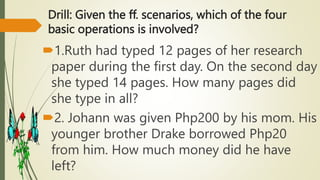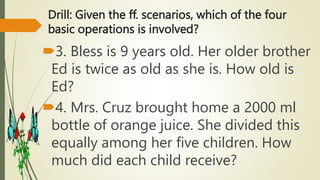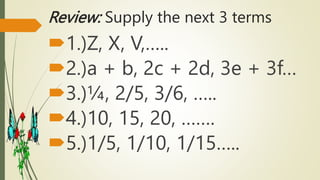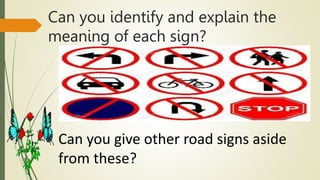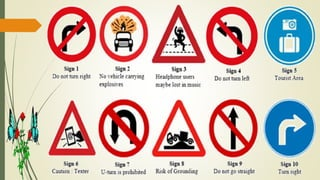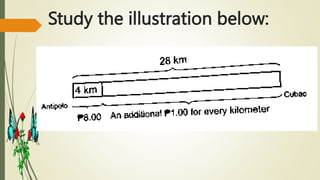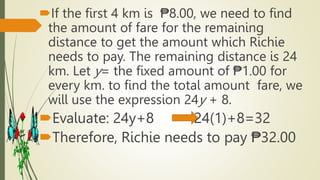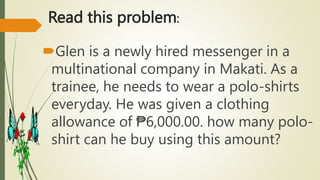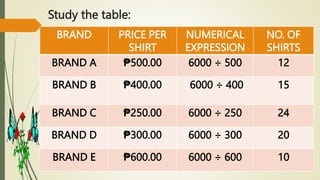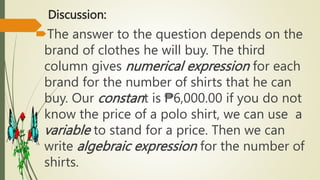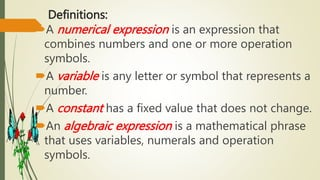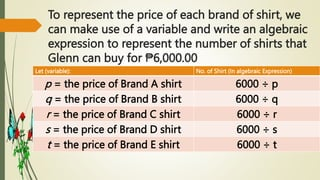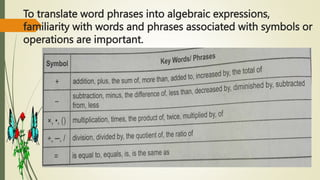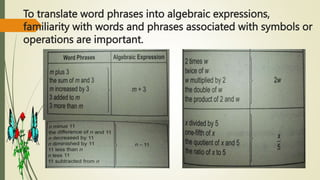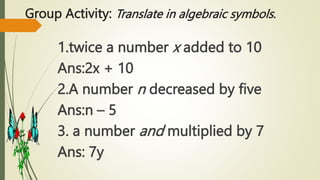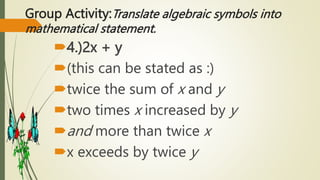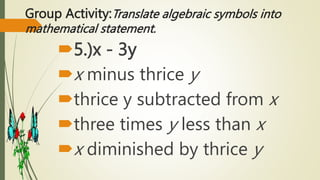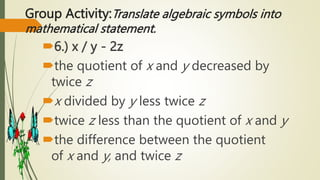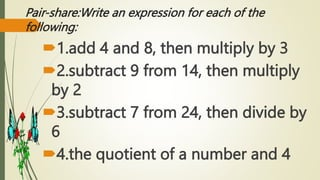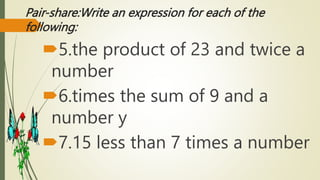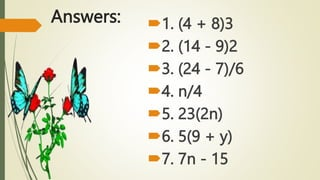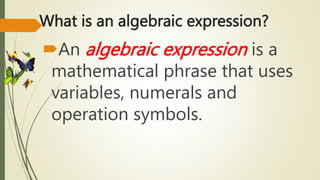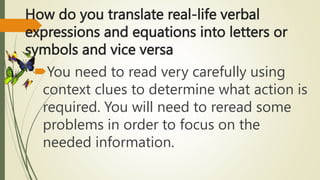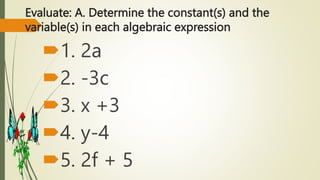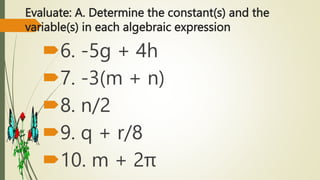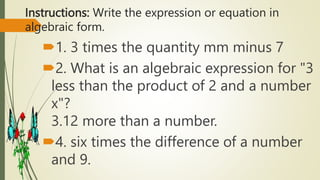1 von 28
Anzeige

### MATH 6 PPT Q3 – Translation Of Real-Life Verbal Expressions And Equations Into Letters Or Symbols.pptx

1. Gives the translation of real-life verbal expressions and equations into letters or symbols and vice versa. Defines a variable in an algebraic expression and equation. Represents quantities in real-life situations using algebraic expressions and equations.
2. Drill: Given the ff. scenarios, which of the four basic operations is involved? 1.Ruth had typed 12 pages of her research paper during the first day. On the second day she typed 14 pages. How many pages did she type in all? 2. Johann was given Php200 by his mom. His younger brother Drake borrowed Php20 from him. How much money did he have left?
3. Drill: Given the ff. scenarios, which of the four basic operations is involved? 3. Bless is 9 years old. Her older brother Ed is twice as old as she is. How old is Ed? 4. Mrs. Cruz brought home a 2000 ml bottle of orange juice. She divided this equally among her five children. How much did each child receive?
4. Review: Supply the next 3 terms 1.)Z, X, V,….. 2.)a + b, 2c + 2d, 3e + 3f… 3.)¼, 2/5, 3/6, ….. 4.)10, 15, 20, ……. 5.)1/5, 1/10, 1/15…..
5. Can you identify and explain the meaning of each sign? Can you give other road signs aside from these?
6. Study the illustration below:
7. If the first 4 km is ₱8.00, we need to find the amount of fare for the remaining distance to get the amount which Richie needs to pay. The remaining distance is 24 km. Let y= the fixed amount of ₱1.00 for every km. to find the total amount fare, we will use the expression 24y + 8. Evaluate: 24y+8 24(1)+8=32 Therefore, Richie needs to pay ₱32.00
8. Read this problem: Glen is a newly hired messenger in a multinational company in Makati. As a trainee, he needs to wear a polo-shirts everyday. He was given a clothing allowance of ₱6,000.00. how many polo- shirt can he buy using this amount?
9. Study the table: BRAND PRICE PER SHIRT NUMERICAL EXPRESSION NO. OF SHIRTS BRAND A ₱500.00 6000 ÷ 500 12 BRAND B ₱400.00 6000 ÷ 400 15 BRAND C ₱250.00 6000 ÷ 250 24 BRAND D ₱300.00 6000 ÷ 300 20 BRAND E ₱600.00 6000 ÷ 600 10
10. Discussion: The answer to the question depends on the brand of clothes he will buy. The third column gives numerical expression for each brand for the number of shirts that he can buy. Our constant is ₱6,000.00 if you do not know the price of a polo shirt, we can use a variable to stand for a price. Then we can write algebraic expression for the number of shirts.
11. Definitions: A numerical expression is an expression that combines numbers and one or more operation symbols. A variable is any letter or symbol that represents a number. A constant has a fixed value that does not change. An algebraic expression is a mathematical phrase that uses variables, numerals and operation symbols.
12. To represent the price of each brand of shirt, we can make use of a variable and write an algebraic expression to represent the number of shirts that Glenn can buy for ₱6,000.00 Let (variable): No. of Shirt (In algebraic Expression) p = the price of Brand A shirt 6000 ÷ p q = the price of Brand B shirt 6000 ÷ q r = the price of Brand C shirt 6000 ÷ r s = the price of Brand D shirt 6000 ÷ s t = the price of Brand E shirt 6000 ÷ t
13. To translate word phrases into algebraic expressions, familiarity with words and phrases associated with symbols or operations are important.
14. To translate word phrases into algebraic expressions, familiarity with words and phrases associated with symbols or operations are important.
15. Group Activity: Translate in algebraic symbols. 1.twice a number x added to 10 Ans:2x + 10 2.A number n decreased by five Ans:n – 5 3. a number and multiplied by 7 Ans: 7y
16. Group Activity:Translate algebraic symbols into mathematical statement. 4.)2x + y (this can be stated as :) twice the sum of x and y two times x increased by y and more than twice x x exceeds by twice y
17. Group Activity:Translate algebraic symbols into mathematical statement. 5.)x - 3y x minus thrice y thrice y subtracted from x three times y less than x x diminished by thrice y
18. Group Activity:Translate algebraic symbols into mathematical statement. 6.) x / y - 2z the quotient of x and y decreased by twice z x divided by y less twice z twice z less than the quotient of x and y the difference between the quotient of x and y, and twice z
19. Pair-share:Write an expression for each of the following: 1.add 4 and 8, then multiply by 3 2.subtract 9 from 14, then multiply by 2 3.subtract 7 from 24, then divide by 6 4.the quotient of a number and 4
20. Pair-share:Write an expression for each of the following: 5.the product of 23 and twice a number 6.times the sum of 9 and a number y 7.15 less than 7 times a number
21. Answers: 1. (4 + 8)3 2. (14 - 9)2 3. (24 - 7)/6 4. n/4 5. 23(2n) 6. 5(9 + y) 7. 7n - 15
22. What is an algebraic expression? An algebraic expression is a mathematical phrase that uses variables, numerals and operation symbols.
23. How do you translate real-life verbal expressions and equations into letters or symbols and vice versa You need to read very carefully using context clues to determine what action is required. You will need to reread some problems in order to focus on the needed information.
24. Evaluate: A. Determine the constant(s) and the variable(s) in each algebraic expression 1. 2a 2. -3c 3. x +3 4. y-4 5. 2f + 5
25. Evaluate: A. Determine the constant(s) and the variable(s) in each algebraic expression 6. -5g + 4h 7. -3(m + n) 8. n/2 9. q + r/8 10. m + 2π
26. Instructions: Write the expression or equation in algebraic form. 1. 3 times the quantity mm minus 7 2. What is an algebraic expression for "3 less than the product of 2 and a number x"? 3.12 more than a number. 4. six times the difference of a number and 9.
27. MERCEDES T. TUNGPALAN Teacher
Anzeige